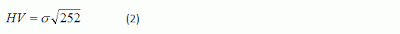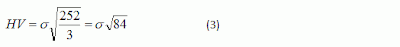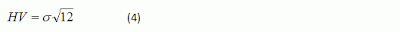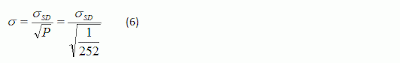I’ve read a few option books.
THANKS... This is probably the most comprehensive "greeks" article/book I’ve read.

Wonderful blog. …..
A wonder wealth of knowledge there. Thanks so much for your kindness in publishing it!

Thank you very much for the most concise and simplest option intro. Highly recommended.

So far, yours is the best blog/site on basic options notes in the web that I have chanced upon.

## Thursday, May 26, 2011

### Historical Volatility – Part 5: How To Annualise Standard Deviation

Go back to Part 4: Understanding Standard Deviation

As mentioned earlier, Historical Volatility is actually a standard deviation. The standard deviation can be calculated using historical price data in terms of daily, weekly, monthly, quarterly or yearly.
Historical Volatility is then expressed in terms of annualised standard deviation of % price returns, so that it can be compared across different stocks, regardless of the stock price and period used for HV calculation.

The formula to annualise the Standard Deviation (that may be calculated using either daily, weekly, monthly, quarterly or yearly) is as follow:Where:
HV = Historical Volatility (annualised)
Sigma = Standard Deviation for a particular time period
T = Number of times (count) of such time periods in a year

So, the value of T in the above formula will depend on the time period of the data used.

In the example used in Part 3, we use daily price returns to calculate standard deviation. Assuming there are 252 trading days in a year, the value of T = 252 / 1 day = 252, because there are 252 times of 1-day period in a year. Hence, we can annualise it by using the following formula:Just for the sake of giving more examples for better understanding of the value of T.
Suppose that 3-day price return data (i.e. the closing prices for every 3 days) is used to calculate the standard deviation. In this case, the value of T = 252 / 3 days = 84, because there are 84 times of 3-day period in a year. Hence, the formula will be:In the case of monthly data is used (i.e. using month-end closing prices), the value of T will be 12 because there are 12 months in a year. Hence, the formula to annualise the monthly data is as follow:For example:
If it is known that the “monthly” standard deviation of Stock ABC’s price returns is 15%, its Historical Volatility will be:Note:
In Wikipedia, the formula to annualise the standard deviation is as follow:
http://en.wikipedia.org/wiki/Volatility_(finance)Where:
Sigma = Annualised Volatility
Sigma SD = Standard Deviation for a particular time period
P = Time period of returns (expressed in terms of year)

To annualise a daily (i.e. 1 day) standard deviation, the value of P will be 1/252 (i.e. 1 day expressed in terms of year). So, the formula will be:This Formula (6) is actually the same as Formula (2), because:I found some people had commented that the formula in Wikipedia is not right. Actually, the formula is right. But we should understand what the logic is and understand the “definition” for the variables. Do compare the definition for T and P, and notice when we should multiply or divide when we want to annualise from daily standard deviation or to convert the annualised standard deviation into daily standard deviation. Just choose one that can make more sense to you.

Continue to Part 6: Interpretation

To view the list of all the series on “Historical Volatility”, please refer to: “More Understanding about HISTORICAL VOLATILITY

Other Learning Resources:
* FREE Trading Educational Videos with Special Feature